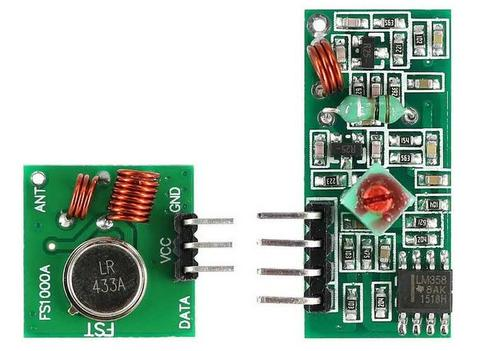# 433MHz RF Wireless Module: Sending Data

433Mhz RF Wireless Transmitter + Receiver for the Arduino and Raspberry Pi. You can use this RF Modules to send wireless data from one Arduino to another.

This tutorial, we'll be sending data from the transmitter to the receiver and we will be using LED as a response on the receiver that the data has been captured.

#### Circuit and Connection#### Transmitter Code

 #include int buttonOne = 11;int buttonTwo = 7;bool state = true;bool state2 = true;char *controller;void setup() {   pinMode(buttonOne, INPUT);   pinMode(buttonTwo, INPUT);   pinMode(9,OUTPUT);   vw_set_ptt_inverted(true); //   vw_set_tx_pin(12);   vw_setup(4000);// speed of data transfer Kbps   digitalWrite(9, LOW);}void loop(){   if(digitalRead(buttonOne) == HIGH){      if(state == true){         controller="Turn on LED" ;         vw_send((uint8_t *)controller, strlen(controller));         vw_wait_tx(); // Wait until the whole message is gone         digitalWrite(9,HIGH);         state = false;      }else {state = true;}   }   if(digitalRead(buttonTwo) == HIGH){      if(state2 == true){         controller="Turn off LED" ;         vw_send((uint8_t *)controller, strlen(controller));         vw_wait_tx(); // Wait until the whole message is gone         digitalWrite(9,LOW);         state2 = false;      }else {state2 = true;}   }}

 #include String inputString = "";void setup(){   Serial.begin(9600);   vw_set_ptt_inverted(true); // Required for DR3100   vw_set_rx_pin(11);   vw_setup(4000); // Bits per sec   pinMode(12, OUTPUT);   vw_rx_start(); // Start the receiver PLL running} void loop(){   uint8_t buf[VW_MAX_MESSAGE_LEN];   uint8_t buflen = VW_MAX_MESSAGE_LEN;   if(vw_get_message(buf, &buflen)) // Non-blocking   {      if(buf=='1'){         digitalWrite(12,1);      }       if(buf=='0'){          digitalWrite(12,0);      }       for (int i = 0; i < buflen; i++)      {         char inchar = char(buf[i]);         inputString += inchar;       }      Serial.println(inputString);      inputString = "";   }}

Test it in an open area first to know if its working.

Done!

Happy Coding!